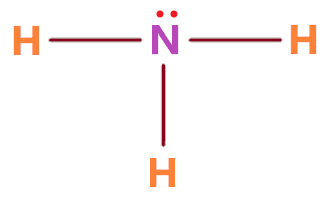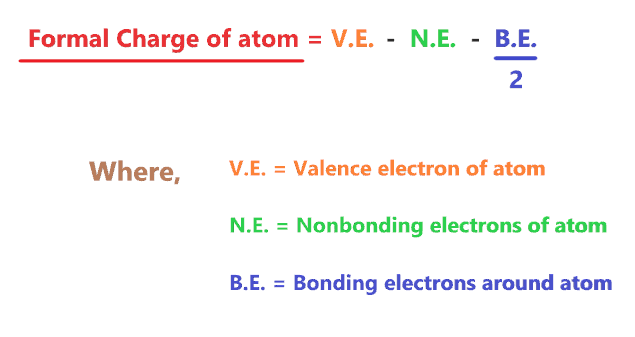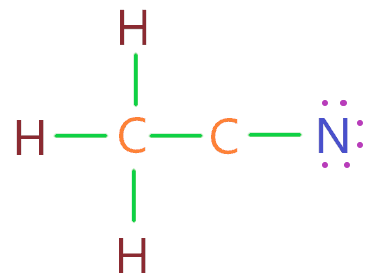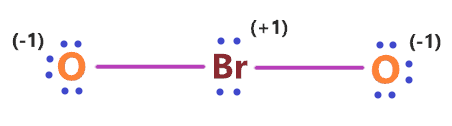# How to draw Lewis structure for any compounds |Lewis dot structure|

Home How to draw Lewis structure?A Lewis structure is also known as the Lewis dot structure is a representation of electrons distribution around the atoms. Using lewis’s structure, we can determine the lone pair and bond pair in the molecule which is eventually helpful in predicting the shape or geometry of the molecule.

How to draw a Lewis structure?

For most of the compounds, the lewis structure is drawn using 5 or 6 simple steps-

1. Calculate the total number of valence electrons in the molecule
2. Choose the central and outer atoms
3. Draw a skeletal structure
4. Complete the octet of outer atoms first
5. Complete the octet of the central atom (make multiple bonds if necessary)
6. Check the formal charge for atoms
Page Contents

## How to Draw Lewis structure for Compounds?

Let’s try to draw the lewis structure for simple compounds, for example – NH3.

To draw the lewis structure of NH3, we will follow five simple steps-

1. Count total valence electron in NH3

First of all, determine the valence electron that is available for drawing the lewis structure because the lewis diagram is all about the representation of valence electrons around atoms.

⇒ Total number of the valence electrons in nitrogen = 5

⇒ Total number of the valence electrons in hydrogen = 1

∴ Total number of valence electrons available for the NH3 Lewis structure = 5 + 1×3 = 8 valence electrons         [∴ NH3 molecule has one nitrogen and three hydrogen atoms]2. Choose a central atom

An atom with a less electronegative value is preferable for the central position in the lewis diagram because they are more prone to share the electrons with surrounding atoms.

It should be noted that “Hydrogen always go outside in lewis diagram” Because, Hydrogen atom can form only one single bond.

Hence, put the nitrogen atom at the central position of the lewis diagram and all three hydrogen atoms outside it.3. Draw Skeletal structure

The skeletal structure is drawn simply by connecting each outer atom to the central atom with a single bond.

In, the NH3 molecule, hydrogen is the outer atom, and nitrogen is the central atom. Hence, joined them as shown in the figure given below.Count the number of valence electrons used in the above structure. There are 3 single bonds used in the above structure, and one single bond means 2 electrons.

Hence, in the above structure, (3 × 2) = 6 valence electrons are used from a total of 8 valence electrons available for drawing the NH3 Lewis structure.

∴ (8 – 6) = 2 valence electrons

So, we are left with only 2 valence electrons.

4. Complete the octet of all atoms

In the 3rd step structure, the hydrogen atoms completed their octet since they have 2 electrons(one single bond means 2 electrons) in their outer shell.

Hydrogen atom only need 2 electrons to fulfill the outer shell.

Now the Nitrogen central atom, in the NH3 molecule, requires a total of 8 electrons to have a full outer shell.

If you look at the 3rd step structure, the nitrogen atom is attached to three single bonds that means it have 6 electrons, so, it just short of 2 electrons.

We already have the remaining 2 valence electrons, hence, put these two electrons on the nitrogen atom to complete its octet as well.In the above structure, we see, that each atom completed its octet comfortably, now, Let’s check the formal charge for the above structure to verify whether it’s stable or not.

5. Check the stability with the help of a formal charge concept

The lesser the formal charge on atoms, the better the stability of the lewis diagram.

To calculate the formal charge on an atom. Use the formula given below-⇒ Formal charge = (valence electrons – nonbonding electrons –   1/2 bonding electrons)

Or to get answer quickly use –

Let’s count the formal charge for the 4th step structure.

For hydrogen atom

⇒ Valence electrons of hydrogen = 1

⇒ Nonbonding electrons on hydrogen = 0

⇒ Bonding electrons around hydrogen (1 single bond) = 2

∴ (1 – 0 – 2/2) = 0 formal charge on the hydrogen atoms.

For nitrogen atom

⇒ Valence electrons of nitrogen = 5

⇒ Nonbonding electrons on nitrogen = 2

⇒ Bonding electrons around nitrogen (3 single bonds) = 6

∴ (5 – 2 – 6/2) = 0 formal charge on the nitrogen central atom.Hence, in the above NH3 lewis structure, all atoms get a formal charge equal to zero.

Therefore, the above lewis dot structure of NH3 (Ammonia) is most stable and appropriate in nature.

That’s all, for most of the simple compounds the lewis structure is drawn by these simple steps.

For examples

Also check –

All these lewis structures given above are drawn by the simple steps as we have already discussed, you can check the process of drawing the lewis structure of these compounds by clicking the links.

## How to Draw Lewis dot structure for Complex compounds?

Now, let’s try to draw the lewis structure for little complex compounds, For example – CO2 molecule.

1. Count total valence electron in CO2

Valence electron of Oxygen = 6          [∴ Periodic group of oxygen = 16 or 6A]

Valence electron of Carbon =          [∴ Periodic group of carbon = 14 or 4A]

Total valence electron available for drawing the CO2 lewis structure = 4 + 2*6 = 16 valence electrons             [∴ CO2 molecule has one carbon and two oxygen atoms]2. Choose a central atom

The electronegativity of the oxygen atom is 3.44 and for the carbon atom, it is 2.55

Clearly, the Carbon atom is less electronegative than Oxygen, therefore, place it at the center of the lewis diagram and put the oxygen atoms on either side of it.3. Draw the skeletal structure

Simply connect both oxygen atoms to the central carbon atom with a single bond.By looking at the above diagram, we come to know that two single bonds are used that contain 4 electrons. (A single bond means 2 electrons)

So, we used 4 electrons from a total of 16 valence electrons that are available for drawing the Lewis structure of CO2.

∴ (16 – 4) = 12 valence electrons

Now we are left with 12 valence electrons.

4. Complete the octet of the outer atom first

As we are left with 12 valence electrons and we have to place these electrons around the outer atom(Oxygen) first to complete its octet rule.

“Octet rule show that atom is most stable when eight electrons present in its valence shell.”

So, oxygen needs 8 electrons around it for coming into the stable zone. Therefore, place the remaining valence electron around oxygen first for completing its octet shell.So, look at the above diagram and see how many valence electrons we used till now and how many are left. Each oxygen has 8 electrons(6 dot electrons + 2 electrons in a single bond), therefore, oxygen atoms completed their octets comfortably.

In the above diagram, 16 valence electrons are used(6 on each oxygen atom + 4 electrons in form of two single bonds).

So, we are left with zero valence electrons.

5. Complete the central atom octet

Now, this is the final step for completing the CO2 lewis dot structure. In this step, we have to complete the central atom(Carbon) octet for its stability.

As carbon needs 8 electrons to complete its octet shell but carbon has only 4 electrons(two single bonds) around it. (Look at 4th step structure).

therefore, the carbon atom needs 4 more electrons to complete its octet.

Also, we have no extra valence electrons left for completing the octet of carbon. So, to overcome this problem, we will take the help of oxygen lone pair electrons.

We will convert the one lone pair of each oxygen atom into a covalent bond.Now look at the above structure and see if the atoms of the CO2 molecule, completed their octet or not.

The carbon central atom has 8 electrons in its valence shell, since, it connected with 2 double bonds. [∴ 1 double bond means 4 electrons].

Also, both oxygen also has 8 electrons, as they are connected with one double bond means 4 electrons + 4 electrons represented as dots.

Yes, both atoms(Carbon and oxygen) have completed their octet rule comfortably as each of them has 8 electrons in the outermost shell.

Now just check the stability of the above structure with the help of the formal charge concept.

6. Check the stability with the help of a formal charge concept

To calculate the formal charge on an atom. Use the formula given below-

⇒ Formal charge = (valence electrons – nonbonding electrons –   1/2 bonding electrons)

We will calculate the formal charge for the 5th step structure.

For carbon atom:

⇒ Valence electrons of carbon = 4

⇒ Nonbonding electrons on carbon = 0

⇒ Bonding electrons around carbon (two double bonds) = 8

∴ (4 – 0 – 8/2) = 0 formal charge on the central carbon atom.

For oxygen atom

⇒ Valence electrons of oxygen = 6

⇒ Nonbonding electrons on oxygen = 4

⇒ Bonding electrons around oxygen (one double bond) = 4

∴ (6 – 4 – 4/2) = 0 formal charge on the oxygen atom.

So, both atoms(carbon and oxygen) get a formal charge equal to zero.Check the lewis structure for these compounds as well-

## How to draw Lewis structure for Large compounds?

Let’s take an example of the CH3CN compound, it is a large and complex compound.

1. Count total valence electron in CH3CN

⇒ Total number of the valence electrons in carbon = 4

⇒ Total number of the valence electrons in nitrogen = 5

⇒ Total number of the valence electrons in hydrogen = 1

∴ Total number of valence electron available for CH3CN lewis structure = 4 + 1(3) + 4 + 5 = 16 valence electrons         [∴CH3CN molecule has two carbon, three hydrogen atom, and one nitrogen]

2. Choose a central atom

In the case of the CH3CN molecule, hydrogen always goes outside as it can share only a maximum of two electrons, from carbon and nitrogen atom, carbon is the less electronegative atom.

Therefore, put the two carbon atoms adjacent to each other in a central position, and spread the three hydrogens around one carbon atom(CH3), and set the nitrogen atom adjacent to other carbon(C≡N).3. Draw the skeletal structure

Now connect each outer atom to the central atom with a single bond.As you see in the above figure, there is a total of 5 single bonds are placed in between the atoms. One single bond contains two electrons, so, these 5 bonds contain a total of 10 valence electrons.

This means we used 10 valence electrons in the above structure from a total of 16 available valence electrons for drawing the CH3CN lewis structure.

∴ (16 – 10) = 6 valence electrons

So, we are left with 6 valence electrons more.

4. Complete octet of outer atoms first

The outer atom in the CH3CN molecule is hydrogen and nitrogen, since, the hydrogen atom only needs two electrons for completing the octet and it has already two electrons because of the single bond attached to it.

So, place the remaining 6 valence electrons around the nitrogen atom to complete its octet.By looking at the above figure, we see nitrogen atom completed its octet as it has 8 valence electrons in the outermost shell, hydrogen atoms also completed their octet as they only need two electrons which are fulfilled by a single bond attached to it.

The left side carbon atom also completed its octet as it is sharing 8 electrons with the help of 4 single bonds, but the right side carbon atom has only 4 electrons(2 single bonds).

So, it needs 4 more valence electrons to complete the octet.

Let’s complete its desire in the next step-

5. Complete octet of the central atom

The only right side carbon atom is left without the octet. To complete the octet of right side carbon, convert the two lone pairs of nitrogen atoms into a covalent bond.As you see in the above figure, we have successfully converted the lone pair of nitrogen atoms into covalent bonds without violating the octet. Now right side carbon atom is also completed its octet as it has 8 electrons to share(one triple bond contains 6 electrons).

So, all the atoms fulfilled the octet comfortably, now just check for the formal charge to know if the above CH3CN lewis structure is stable or not.

6. Check the stability with the help of a formal charge concept

To determine the formal charge on an atom. Use the formula given below-

⇒ Formal charge = (valence electrons – lone pair electrons –  1/2 bonded pair electrons)

For hydrogen atom:

⇒ Valence electrons of hydrogen = 1

⇒ Lone pair electrons on hydrogen = 0

⇒ Shared pair electrons around hydrogen(1 single bond) = 2

∴ (1 – 0 – 2/2) = 0 formal charge on all hydrogen atoms.

For nitrogen atom

⇒ Valence electrons of nitrogen = 5

⇒ Lone pair electrons on nitrogen = 2

⇒ Shared pair electrons around nitrogen (1 triple bond) = 6

∴ (5 – 2 – 6/2) = o formal charge on the nitrogen atom.

For the left side carbon atom

⇒ Valence electrons of carbon = 4

⇒ Lone pair electrons on carbon = 0

⇒ Shared pair electrons around carbon (4 single bonds) = 8

∴ (4 – 0 – 8/2) = o formal charge on the left side carbon atom.

For the right side carbon atom

⇒ Valence electrons of carbon = 4

⇒ Lone pair electrons on carbon = 0

⇒ Shared pair electrons around carbon (one triple bond and one single bond) = 8

∴ (4 – 0 – 8/2) = o formal charge on the right side carbon atom.

∴ All atoms in the CH3CN molecule get a formal charge equal to zero.Check the lewis structure for these compounds as well-

## How to draw a Lewis structure for Charged molecules?

The charged molecule is also called polyatomic ions, their net charge is not zero, they have ions, hence, learning the process of drawing the Lewis structure for these types of molecules is very important.

Let’s take an example of BrO2 molecule, it has one negative charge.

1. Count total valence electron in BrO2

⇒ Total number of the valence electrons in oxygen = 6

⇒ Total number of the valence electrons in bromine = 7

∴ Total number of valence electron available for the BrO2 lewis structure = 7 + 6(2) + 1 = 20 valence electrons         [∴ one bromine, two oxygen and one negative ion in BrO2 that also count as a one valence electron]2. Choose a central atom

A bromine atom(2.96) is less electronegative than an oxygen atom(3.44), hence, putting the bromine in the central position of the lewis diagram and oxygen as terminal atoms.3. Draw the skeletal structure

Now just attach the single bond for connecting each outer atom(oxygen) to the central atom(bromine).Count the valence electron we used to draw the above structure. A single bond means two electrons and in the above structure, two single bonds are used.

Therefore, (2 single bonds × 2 electrons) = 4 valence electrons are used in the above structure from a total of 20 valence electrons available for the BrO2 lewis structure.

∴ (20 – 4) = 16 valence electrons

Hence, we are left with 16 valence electrons more.

4. Complete the octet of the outer atom first

Here’s we need to put our remaining valence electron over outer atoms first to complete their octet.

In the case of the BrO2 molecule, oxygen atoms are outer atoms and they need 8 electrons to complete their outer shell.As you see in the above structure, we put the 6 electrons represented as dots on both oxygens. Now Oxygen atoms completed their octet, since, both have 8 electrons(6 represented as dots + 2 electrons in a single bond).

Again count the total valence electrons used in the above structure.

In the above structure, (2 single bonds mean 4 electrons + 12 electrons represented as dots) = 16 valence electrons are used from the total of 20 electrons available for BrO2- lewis structure.

∴ (20 – 16) = 4 valence electrons

Now we are left with only 4 valence electrons.

5. Complete the octet of the central atom

Bromine is the central atom in BrO2 molecule. And we have 4 remaining valence electrons, hence, put these remaining valence electrons over the Bromine central atom.If you look at the above structure, we see that all atoms(oxygen and bromine) completed their octet comfortably as each of them has 8 valence electrons(electrons represented as dots + electrons in a single bond) in their outer shell.

Also, we used all 20 valence electrons that are available for BrO2.

Now we just need to check the stability of the above structure through the formal charge concept.

6. Check the stability with the help of a formal charge concept

The structure with the formal charge close to zero or zero is the best and most stable lewis structure.

To calculate the formal charge on an atom. Use the formula given below-

⇒ Formal charge = (valence electrons – Non bonding electrons –  1/2 bonding electrons)

We will calculate the formal charge on the 5th step structure to verify its stability.

For Bromine atom –

⇒ Valence electron of bromine = 7

⇒ Nonbonding electrons on bromine = 4

⇒ Bonding electrons around bromine = 4 (two single bonds)

∴ Formal charge on bromine atom = (7 – 4 – 4/2) = +1

For oxygen atom –

⇒ Valence electron of oxygen = 6

⇒ Nonbonding electrons on oxygen = 6

⇒ Bonding electrons around oxygen = 2 (one single bond)

∴ Formal charge on oxygen atom = (6 – 6 – 2/2) = -1The above structure is not stable as it has an uneven formal charge. We have to lower the formal charge of the above structure.

To reduce the formal charge, We have to convert the one lone pair of oxygen atoms to form a double bond with the central atom.

Note: The bromine atom is exceptional to the octet rule as it can hold more than 8 electrons in its outermost shell. It is also called an expanded octet.

Expanded octet: A case where an atom shares more than eight electrons with its bonding partners.As you see, we reduced the formal charges, and the overall formal charge in the above figure is -1. Since, BrO2 has one negative ion as well, therefore, the entire structure should have a -1 formal charge.As BrO2 molecule contains one negative ion also, so, we need to put the bracket around the BrO2 lewis structure and show a negative ion outside the bracket.

Let’s check the lewis structure for these charged molecules as well-

## How to draw a Lewis dot structure for ionic compounds?

The lewis diagram for an ionic compound is formed with a different approach than the normal procedure we used for drawing the compounds like NH3, BF3, BrF5, etc.

For example – NaCl is an ionic compound, its making of the lewis diagram is different than covalent compounds.

Check thisHow to draw the lewis structure for NaCl

## Summary

Lewis structure or Lewis dot structure is drawn by using 5 or 6 steps.

• Calculate the total number of valence electrons in the molecule
• Choose the central and outer atoms
• Draw a skeletal structure
• Complete the octet of outer atoms first
• Complete the octet of the central atom (make multiple bonds if necessary)
• Check the formal charge for atoms
Share it...